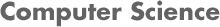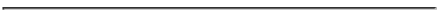### CS 4445 Data Mining and Knowledge Discovery in Databases - B Term 2010  Project 1: Data Exploration and Data Pre-processing

#### PROF. CAROLINA RUIZ

DUE DATE: Friday, Nov. 5, 2010 at the beginning of class (11:00 am)
** This is an individual project **#### PROJECT DESCRIPTION

The purpose of this project is multi-fold:

#### PROJECT ASSIGNMENT

Written Report: Your written report should consist of your answers to each of the parts in the assignment below.

Assignment:

1. Weka and Dataset.

1. Download and install the developer version of the Weka system as described in the Course Webpage. Determine the name/path of the directory created to store the Weka files (e.g., C:\Program Files\Weka-3-5\). We'll call that directory WekaDirectory in the remaining of this project description.

2. The KDD Cup 1999 Data Set contains about 5 million data instances. In this project we will use 10% of this dataset contained in kddcup.data_10_percent.gz. You need to download this 10% file and "gunzip" it. The resulting file is a data file in CSV (comma separated values) format. You can use .csv as the file extension, and look at this file using Excel or any other program you want to use for this purpose. Note that the target classification attribute (let's call it attack_type) is nominal. Its values appear in the first line of kddcup.names), and it is the last column of kddcup.data_10_percent.gz.

3. Convert the dataset to the arff format. For this you can either use any tools provided by Weka, or you can make the conversion outside the Weka system using other tools (e.g., a word editor, Excel, etc.). Create a kddcup.data_10_percent.arff file with the converted dataset.

4. Include in your report the header of your kddcup.data_10_percent.arff file together with the 10 first data instances of the dataset. (Do NOT include the full dataset - Just the first 10 data instances.)

5. Load this dataset into Weka by opening your arff dataset from the "Explorer" window in Weka. Increase the memory available to Weka as needed, but if this dataset still doesn't fit in main memory, remove as many instances from the end of your arff file as you need (but not more!) until it fits in memory.

2. Data Exploration. See Chapter 3 of the textbook. Use the full 10% dataset and Excel, matlab, your own code, Weka (if you can upload the full 10% dataset into Weka), or other software, to complete the following parts. Please state in your report which tool from the above list you used.

1. For each of the discrete attributes (denoted as "symbolic" in kddcup.names), including the target classification attribute:
1. Calculate the frequency and modes of the attribute.
2. Provide a graphical depiction of the distribution of the target classification attribute (attack_type) for each of the values of the attribute under consideration.

2. For each of the continuous attributes (denoted as "continuous" in kddcup.names):
1. Calculate the percentiles (in increments of 10, as in Table 3.2 of the textbook, page 101), mean, median, range, and variance of the attribute.
2. Plot a histogram of the attribute using 10 or 20 bins (you choose the best value for each attribute). For examples, see Figures 3.7 and 3.8 in the textbook, page 113.
3. Plot a graph in which the X axis corresponds to the classification target (attach_type), the Y axis is the continuous attribute under consideration, and each point (x,y) in the plot corresponds to the value of the targe attribute (i.e., x) and the value of the attribute under consideration (i.e., y) of the data instance. For example, if the attribute under consideration is "duration", the plot point corresponding to the first data instance of the dataset is (0, normal).

3. For the set of continuous attributes, calculate the covariance matrix and the correlation matrix of these attributes. If you had to remove 5 continuous attributes from the dataset based on these two matrices, which attributes would you remove and why? Explain your answer.

4. Convert the target attribute (attack_type) into a boolean attribute attack? with value 0 if attack_type="normal", and 1 if attack_type is different from "normal". Calculate the frequency and mode of the new attribute.

3. Data Preprocessing. See Chapter 2 of your textbook. Upload the 10% dataset into Weka as described above. Use the new attack? attribute (instead of the original attack_type as the classification target. We'll refer to this dataset as the "input dataset" below.

1. Sampling.

1. Use Weka's unsupervised Resample filter to obtain a 50% subsample of the input dataset without replacement. Include in your report the distribution of the attack? attribute in the subsample.

2. Use Weka's supervised Resample filter to obtain a 50% subsample of the input dataset without replacement. Include in your report the distribution of the attack? attribute in the subsample.

3. Are the above two distributions different? If so, why?

2. Attribute Discretization.

1. Use Weka's unsupervised Discretize filter to discretize the continuous attribute "duration" of the input dataset into 10 bins using equal frequency (i.e., useEqualFrequency=True). Include the results in your report, as well as the distribution of the attack? attribute in each of the bins.

2. Use Weka's unsupervised Discretize filter to discretize the continuous attribute "duration" of the input dataset into 10 bins using equal width (i.e., useEqualFrequency=False). Include the results in your report, as well as the distribution of the attack? attribute in each of the bins.

3. Use Weka's supervised Discretize filter to discretize the continuous attribute "duration" of the input dataset with respect to the class attribute. Include the results in your report, as well as the distribution of the attack? attribute in each of the resulting bins.

4. Weka Code. Find the Java code that implements the unsupervised discretization filter in the directories that contain the Weka files. You can find the Weka code in a file called "weka-src.jar", which should be located in the directory where Weka was installed. This "weka-src.jar" file is a zip file. Hence you need to winzip or unzip it to extract its contents. Inside, you will find the .java files that implement Weka. Include the code implementing this filter in your report, and describe the algorithm followed by this code when doing unsupervised equal-frequency discretization (i.e., useEqualFrequency=True) in your own words.

3. Dimensionality Reduction. Apply Principal Components Analysis to reduce the dimensionality of the input dataset. For this, use Weka's PrincipalComponents unsupervised filter. Include in your report the linear combinations that define the first two new attributes(= components) obtained.

4. Feature Selection. Apply Correlation Based Feature Selection (see Witten's and Frank's textbook slides - Chapter 7 Slides 5-6) to the input dataset. For this, use Weka's CfsSubsetEval available under the Select attributes tab with default parameters. Include in your report which attributes were selected by this method. Also, what can you observe about these selected attributes with respect to the covariance matrix and the correlation matrix you computed for part 2.2.3 above? Were the 5 attributes you chose to remove in part 2.2.3 above kept or removed by CfsSubsetEval?

#### REPORTS AND DUE DATE

Hand in a hardcopy of your written report at the beginning of class the day the project is due. We will discuss the results from the project during class so be prepared to give an oral presentation.